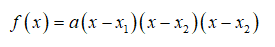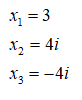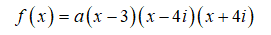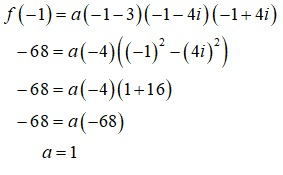# Find an​ nth-degree polynomial function with real coefficients satisfying the given conditions. If you are using a graphing​ utility, use it to graph the function and verify the real zeros and the given function value.n=33 and 4i are zerosf(-1)=-68

Question
6 views
Find an​ nth-degree polynomial function with real coefficients satisfying the given conditions. If you are using a graphing​ utility, use it to graph the function and verify the real zeros and the given function value.
n=3
3 and 4i are zeros
f(-1)=-68
check_circle

Step 1

We write the general form for a 3 degree polynomial function as,From the data provided, since complex roots occur in pairs, 4i and -4i will be the two imaginary roots.Subsitute in the expression for f(x).Step 2

Substitute x=-1...

### Want to see the full answer?

See Solution

#### Want to see this answer and more?

Solutions are written by subject experts who are available 24/7. Questions are typically answered within 1 hour.*

See Solution
*Response times may vary by subject and question.
Tagged in